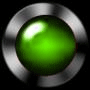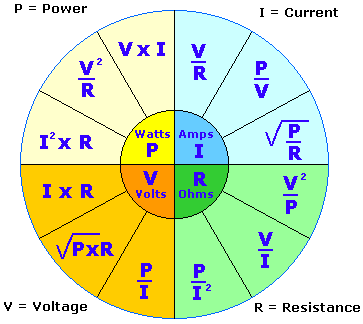Power and unitsForce = a push or Pull                                   measured in Newtons Work = Force over a Distance                     measured in  Joules     ( other wise called energy or Newton meters) Power = the rate at which Energy is used     measured in Watts Power = V x I example: A 240 V motor draws 10A under load, what is the power rating? P= V x I = 2400 watts or 2.4Kw (kilowatts) In electrical terms power is rated in watts and some motors are still rated in horse power (HP) 746 watts = 1 Horse Power unit example: the above motor would be rated as 2400w/750w/hp = 3.2 HP 1000 watts = 1 Kilowatt   kW 1000 000 w = 1megawatt MW .001 watts  = 1milliwatt    mWPOWER CALCULATOR simulator 1000 volts = 1 kilovolt (kV) not likely to encounter this in electronics .001 amps = 1 milliamp (mA) a very common term in electronics